# DirectionalSampling¶

class DirectionalSampling(*args)

Directional simulation.

Refer to Directional Simulation.

Available constructors:

DirectionalSampling(event=ot.Event())

DirectionalSampling(event, rootStrategy, samplingStrategy)

Parameters:
eventRandomVector

Event we are computing the probability of.

rootStrategyRootStrategy

Strategy adopted to evaluate the intersections of each direction with the limit state function and take into account the contribution of the direction to the event probability. By default, rootStrategy = ot.RootStrategy(ot.SafeAndSlow()).

samplingStrategySamplingStrategy

Strategy adopted to sample directions. By default, samplingStrategy=ot.SamplingStrategy(ot.RandomDirection()).

Notes

Using the probability distribution of a random vector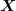, we seek to evaluate the following probability: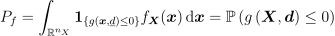Here,is a random vector,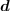a deterministic vector,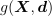the function known as limit state function which enables the definition of the event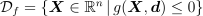.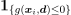describes the indicator function equal to 1 if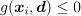and equal to 0 otherwise.

The directional simulation method is an accelerated sampling method. It implies a preliminary iso-probabilistic transformation, as for FORM and SORM methods; however, it remains based on sampling and is thus not an approximation method. In the transformed space, the (transformed) uncertain variables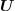are independent standard gaussian variables (mean equal to zero and standard deviation equal to 1).

Roughly speaking, each simulation of the directional simulation algorithm is made of three steps. For the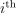iteration, these steps are the following:

• Let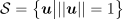. A point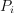is drawn randomly on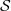according to an uniform distribution.

• In the direction starting from the origin and passing through, solutions of the equation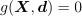(i.e. limits of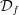) are searched. The set of values ofthat belong tois deduced for these solutions: it is a subset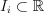.

• Then, one calculates the probability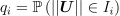. By property of independent standard variable,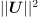is a random variable distributed according to a chi-square distribution, which makes the computation effortless.

Finally, the estimate of the probability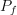after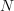simulations is the following: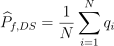Examples

>>> import openturns as ot
>>> ot.RandomGenerator.SetSeed(0)
>>> myFunction = ot.SymbolicFunction(['E', 'F', 'L', 'I'], ['-F*L^3/(3*E*I)'])
>>> myDistribution = ot.Normal([50.0, 1.0, 10.0, 5.0], [1.0]*4, ot.IdentityMatrix(4))
>>> # We create a 'usual' RandomVector from the Distribution
>>> vect = ot.RandomVector(myDistribution)
>>> # We create a composite random vector
>>> output = ot.CompositeRandomVector(myFunction, vect)
>>> # We create an Event from this RandomVector
>>> myEvent = ot.ThresholdEvent(output, ot.Less(), -3.0)
>>> # We create a DirectionalSampling algorithm
>>> myAlgo = ot.DirectionalSampling(myEvent, ot.MediumSafe(), ot.OrthogonalDirection())
>>> myAlgo.setMaximumOuterSampling(150)
>>> myAlgo.setBlockSize(4)
>>> myAlgo.setMaximumCoefficientOfVariation(0.1)
>>> # Perform the simulation
>>> myAlgo.run()
>>> print('Probability estimate=%.6f' % myAlgo.getResult().getProbabilityEstimate())
Probability estimate=0.169716


Methods

 Draw the probability convergence at a given level. Accessor to the block size. Accessor to the object's name. Accessor to the convergence strategy. Accessor to the event. Accessor to the object's id. Accessor to the maximum coefficient of variation. Accessor to the maximum sample size. Accessor to the maximum standard deviation. Accessor to the object's name. Accessor to the results. Get the root strategy. Get the direction sampling strategy. Accessor to the object's shadowed id. Accessor to verbosity. Accessor to the object's visibility state. Test if the object is named. Test if the object has a distinguishable name. Launch simulation. setBlockSize(blockSize) Accessor to the block size. setConvergenceStrategy(convergenceStrategy) Accessor to the convergence strategy. Accessor to the maximum coefficient of variation. setMaximumOuterSampling(maximumOuterSampling) Accessor to the maximum sample size. Accessor to the maximum standard deviation. setName(name) Accessor to the object's name. Set up a progress callback. setRootStrategy(rootStrategy) Set the root strategy. setSamplingStrategy(samplingStrategy) Set the direction sampling strategy. Accessor to the object's shadowed id. setStopCallback(*args) Set up a stop callback. setVerbose(verbose) Accessor to verbosity. setVisibility(visible) Accessor to the object's visibility state.
__init__(*args)
drawProbabilityConvergence(*args)

Draw the probability convergence at a given level.

Parameters:
levelfloat, optional

The probability convergence is drawn at this given confidence length level. By default level is 0.95.

Returns:
graph

probability convergence graph

getBlockSize()

Accessor to the block size.

Returns:
blockSizeint

Number of terms in the probability simulation estimator grouped together. It is set by default to 1.

getClassName()

Accessor to the object’s name.

Returns:
class_namestr

The object class name (object.__class__.__name__).

getConvergenceStrategy()

Accessor to the convergence strategy.

Returns:
storage_strategyHistoryStrategy

Storage strategy used to store the values of the probability estimator and its variance during the simulation algorithm.

getEvent()

Accessor to the event.

Returns:
eventRandomVector

Event we want to evaluate the probability.

getId()

Accessor to the object’s id.

Returns:
idint

Internal unique identifier.

getMaximumCoefficientOfVariation()

Accessor to the maximum coefficient of variation.

Returns:
coefficientfloat

Maximum coefficient of variation of the simulated sample.

getMaximumOuterSampling()

Accessor to the maximum sample size.

Returns:
outerSamplingint

Maximum number of groups of terms in the probability simulation estimator.

getMaximumStandardDeviation()

Accessor to the maximum standard deviation.

Returns:
sigmafloat,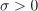Maximum standard deviation of the estimator.

getName()

Accessor to the object’s name.

Returns:
namestr

The name of the object.

getResult()

Accessor to the results.

Returns:
resultsSimulationResult

Structure containing all the results obtained after simulation and created by the method run().

getRootStrategy()

Get the root strategy.

Returns:
strategyRootStrategy

getSamplingStrategy()

Get the direction sampling strategy.

Returns:
strategySamplingStrategy

Accessor to the object’s shadowed id.

Returns:
idint

Internal unique identifier.

getVerbose()

Accessor to verbosity.

Returns:
verbosity_enabledbool

If True, the computation is verbose. By default it is verbose.

getVisibility()

Accessor to the object’s visibility state.

Returns:
visiblebool

Visibility flag.

hasName()

Test if the object is named.

Returns:
hasNamebool

True if the name is not empty.

hasVisibleName()

Test if the object has a distinguishable name.

Returns:
hasVisibleNamebool

True if the name is not empty and not the default one.

run()

Launch simulation.

Notes

It launches the simulation and creates a SimulationResult, structure containing all the results obtained after simulation. It computes the probability of occurrence of the given event by computing the empirical mean of a sample of size at most outerSampling * blockSize, this sample being built by blocks of size blockSize. It allows one to use efficiently the distribution of the computation as well as it allows one to deal with a sample size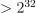by a combination of blockSize and outerSampling.

setBlockSize(blockSize)

Accessor to the block size.

Parameters:
blockSizeint,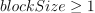Number of terms in the probability simulation estimator grouped together. It is set by default to 1.

Notes

For Monte Carlo, LHS and Importance Sampling methods, this allows one to save space while allowing multithreading, when available we recommend to use the number of available CPUs; for the Directional Sampling, we recommend to set it to 1.

setConvergenceStrategy(convergenceStrategy)

Accessor to the convergence strategy.

Parameters:
storage_strategyHistoryStrategy

Storage strategy used to store the values of the probability estimator and its variance during the simulation algorithm.

setMaximumCoefficientOfVariation(maximumCoefficientOfVariation)

Accessor to the maximum coefficient of variation.

Parameters:
coefficientfloat

Maximum coefficient of variation of the simulated sample.

setMaximumOuterSampling(maximumOuterSampling)

Accessor to the maximum sample size.

Parameters:
outerSamplingint

Maximum number of groups of terms in the probability simulation estimator.

setMaximumStandardDeviation(maximumStandardDeviation)

Accessor to the maximum standard deviation.

Parameters:
sigmafloat,Maximum standard deviation of the estimator.

setName(name)

Accessor to the object’s name.

Parameters:
namestr

The name of the object.

setProgressCallback(*args)

Set up a progress callback.

Can be used to programmatically report the progress of a simulation.

Parameters:
callbackcallable

Takes a float as argument as percentage of progress.

Examples

>>> import sys
>>> import openturns as ot
>>> experiment = ot.MonteCarloExperiment()
>>> X = ot.RandomVector(ot.Normal())
>>> Y = ot.CompositeRandomVector(ot.SymbolicFunction(['X'], ['1.1*X']), X)
>>> event = ot.ThresholdEvent(Y, ot.Less(), -2.0)
>>> algo = ot.ProbabilitySimulationAlgorithm(event, experiment)
>>> algo.setMaximumOuterSampling(100)
>>> algo.setMaximumCoefficientOfVariation(-1.0)
>>> def report_progress(progress):
...     sys.stderr.write('-- progress=' + str(progress) + '%\n')
>>> algo.setProgressCallback(report_progress)
>>> algo.run()

setRootStrategy(rootStrategy)

Set the root strategy.

Parameters:
strategyRootStrategy

setSamplingStrategy(samplingStrategy)

Set the direction sampling strategy.

Parameters:
strategySamplingStrategy

Accessor to the object’s shadowed id.

Parameters:
idint

Internal unique identifier.

setStopCallback(*args)

Set up a stop callback.

Can be used to programmatically stop a simulation.

Parameters:
callbackcallable

Returns an int deciding whether to stop or continue.

Examples

Stop a Monte Carlo simulation algorithm using a time limit

>>> import openturns as ot
>>> experiment = ot.MonteCarloExperiment()
>>> X = ot.RandomVector(ot.Normal())
>>> Y = ot.CompositeRandomVector(ot.SymbolicFunction(['X'], ['1.1*X']), X)
>>> event = ot.ThresholdEvent(Y, ot.Less(), -2.0)
>>> algo = ot.ProbabilitySimulationAlgorithm(event, experiment)
>>> algo.setMaximumOuterSampling(10000000)
>>> algo.setMaximumCoefficientOfVariation(-1.0)
>>> timer = ot.TimerCallback(0.1)
>>> algo.setStopCallback(timer)
>>> algo.run()

setVerbose(verbose)

Accessor to verbosity.

Parameters:
verbosity_enabledbool

If True, make the computation verbose. By default it is verbose.

setVisibility(visible)

Accessor to the object’s visibility state.

Parameters:
visiblebool

Visibility flag.

## Examples using the class¶Use the Directional Sampling Algorithm

Use the Directional Sampling AlgorithmAxial stressed beam : comparing different methods to estimate a probability

Axial stressed beam : comparing different methods to estimate a probability